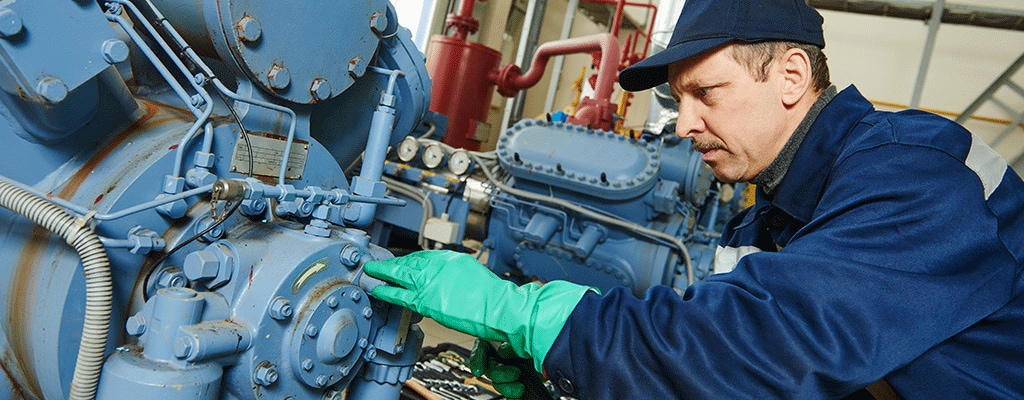### Compound Pressure Transducers

Measure negative and positive gauge pressure.

Different terms are used in the compressor industry when referring to pressure transducers that measure from negative to positive gauge pressure. Measuring both suction and discharge, a compound pressure transducer provides a linear output signal from full vacuum through zero pressure up to the full scale pressure. A pressure transducer with a pressure range of -14.7 to 30 PSIG can be referred to as a compound pressure transducer, 30V30 (referring to -30" of mercury) transducer, or a vacuum to 30 PSIG sensor.  While the terminology differs, it can refer to the same product. Calculating the offset of the pressure transducer as it should read with no pressure (or the pressure on the bench) can be confusing without writing it down.  Here are a couple of examples to help walk you through the process for both 4-20mA output signals and voltage signals. The same principles apply to other pressures and output signals.  We started with a pressure measurement range of -14.7 to 15 PSI to help visualize how the calculation should work.  While 0 PSI is close to the mid-point between the output, it is not exact; a few extra steps lead us to the right reading.

#### 4-20mA Example

AST4300AV0015P4N1000
AST4300 Non-Incendive Pressure Transmitter
1/4" NPT Male process connection
-14.7 to 15 PSIG
4-20mA output signal
Conduit with 6 foot cable
316L stainless steel
First, you want to find out how many milliamps per PSI.
You will be measuring 14.7 + 15 = 29.7 PSI over the full scale pressure range
20mA - 4mA = 16 mA of span
16mA / 29.7 psi = 0.5389 mA / PSI
Next, the "0" PSI pressure is going to be 14.7 psi above the 4mA output, because the pressure transducer is scaled from -14.7 to full scale. 14.7 PSI x 0.539 mA/PSI = 7.923 mA
Finally ADD back in the 4mA for the zero offset (since the output starts @ 4mA and not 0 mA):
4 + 7.923 mA = 11.923 mA @ 0 PSI

4mA = -14.7 PSI
11.923 mA is the theoretical output of 0 PSI
20mA = 15 PSI full scale pressure

### 4-20mA Popular Compound Pressures

Pressure Range Approximate Reading at Zero Pressure
-14.7 to 15 PSI 11.92 mA
-14.7 to 30 PSI 8.93 mA
-14.7 to 50 PSI 7.48 mA
-14.7 to 100 PSI 6.05 mA
-14.7 to 185 PSI 5.18 mA
-14.7 to 300 PSI 4.75 mA
-14.7 to 500 PSI 4.46 mA

#### Voltage Example

AST4000KV0185P1F0143
AST4000 Industrial Pressure Transducer
SAE 4 female process connection with Schraeder Pin
-14.7 to 185 PSIS
0.5-4.5V ratiometric output signal
Packard Metripack 150
17-4 PH stainless steel

You will be measuring 14.7 + 185 = 199.7 PSI over the full scale pressure range
4.5V - 0.5V = 4V of span
4V / 199.7 PSI = 0.020V / PSI
Next, the "0" PSI pressure is going to be 14.7 psi above the 0.5V output, because the pressure transducer is scaled from -14.7 to full scale. 14.7 PSI x 0.020V / PSI = 0.294V
Finally ADD back in the 0.5V for the zero offset (since the output starts @ 0.5V and not 0 V):
0.5 + 0.294441V = 0.794V @ 0 PSI

0.5V = -14.7 PSI
0.794V is the theoretical output of 0 PSI
4.5V = 185 PSI full scale pressure

### 0.5-4.5V Ratiometric Compound Pressures

Pressure Range Approximate Reading at Zero Pressure
-14.7 to 15 PSI 2.48V
-14.7 to 30 PSI  1.73V
-14.7 to 50 PSI 1.52V
-14.7 to 100 PSI 1.01V
-14.7 to 185 PSI 0.80V
-14.7 to 300 PSI 0.69V
-14.7 to 500 PSI 0.61V

As the pressure increases, the amount of output signal for the compound range between -14.7 and 0 PSI decreases.  Once sensor error, such as zero offset is calculated, the ability to accurately measure the vacuum portion of the transducer diminishes.  The pressures in the above charts are the most commonly calculated, although it is possible to calibrate higher pressures with compound pressure ranges.  Other pressure units, such as Bar and kg/cm2, are also available using the same methodology.